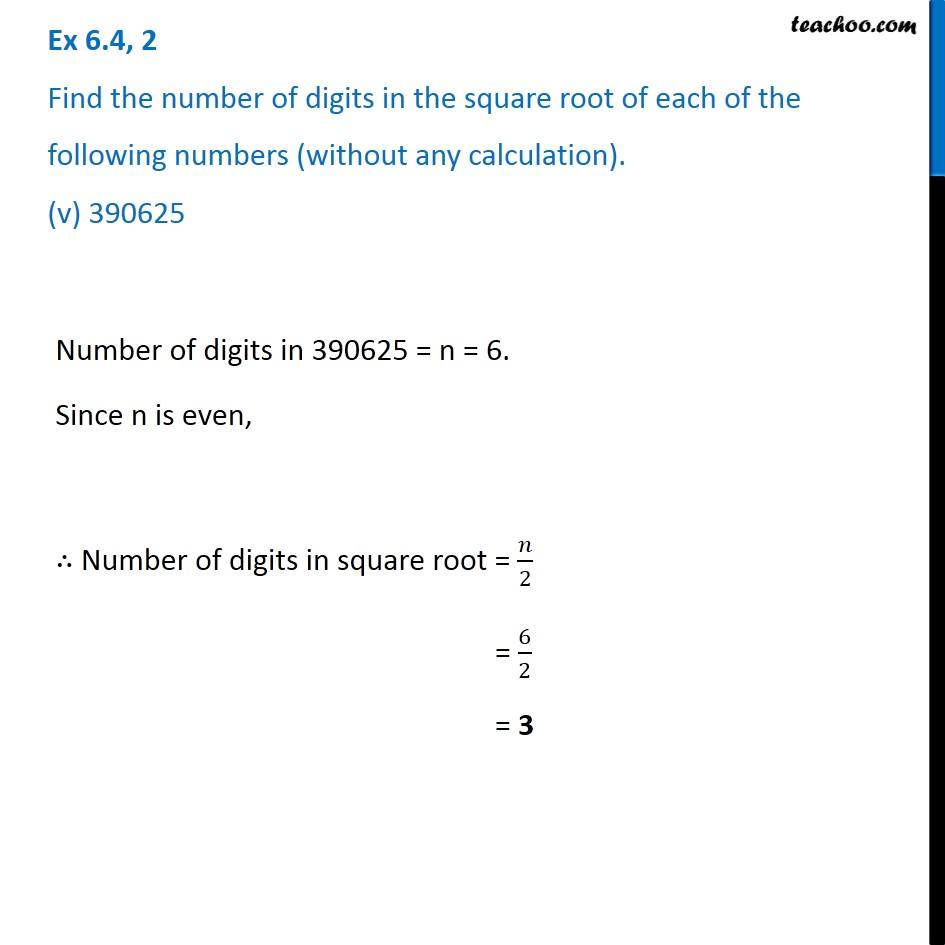Ex 6.4

Chapter 6 Class 8 Squares and Square Roots
Serial order wise### Transcript

Ex 6.4, 2 Find the number of digits in the square root of each of the following numbers (without any calculation). (v) 390625 Number of digits in 390625 = n = 6. Since n is even, ∴ Number of digits in square root = 𝑛/2 = 6/2 = 3